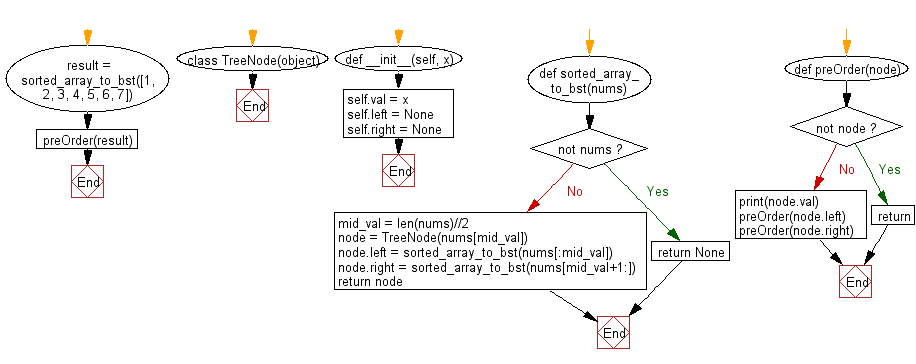﻿ Python Binary Search Tree: Create a Balanced Binary Search Tree (BST) using an sorted array - w3resource

# Python Binary Search Tree: Create a Balanced Binary Search Tree (BST) using an sorted array

## Python Binary Search Tree: Exercise-1 with Solution

Write a Python program to create a Balanced Binary Search Tree (BST) using an array of elements where array elements are sorted in ascending order.

Pictorial Presentation:Sample Solution:

Python Code:

``````class TreeNode(object):
def __init__(self, x):
self.val = x
self.left = None
self.right = None

def sorted_array_to_bst(nums):

if not nums:
return None
mid_val = len(nums)//2
node = TreeNode(nums[mid_val])
node.left = sorted_array_to_bst(nums[:mid_val])
node.right = sorted_array_to_bst(nums[mid_val+1:])
return node

def preOrder(node):
if not node:
return
print(node.val)
preOrder(node.left)
preOrder(node.right)

result = sorted_array_to_bst([1, 2, 3, 4, 5, 6, 7])
preOrder(result)
```
```

Sample Output:

```4
2
1
3
6
5
7
```

Flowchart:Python Code Editor:

What is the difficulty level of this exercise?

Test your Programming skills with w3resource's quiz.

﻿

## Python: Tips of the Day

Joining Collection:

```name = ["Owen", "Eddie"]
print(" ".join(name))
```
`Owen Eddie`

We are closing our Disqus commenting system for some maintenanace issues. You may write to us at reach[at]yahoo[dot]com or visit us at Facebook